# Fourier series and sketch the waveform

Member warned about posting with no effort

## Homework Statement

Sketch the waveform defined below and explain how you would obtain its Fourier series:
f(wt) = 0 for 0 ≤wt ≤pi/2 (w=omega)
f(wt) = Vsin(wt) for pi/2 ≤wt ≤pi
f(wt) = 0 for pi ≤wt ≤3pi/2
f(wt) = Vsin(wt) for 3pi/2 ≤wt ≤2pi
Develop the analysis as far as you are able.

## The Attempt at a Solution

Any help how to start this is appreciated..

gneill
Mentor
You need to make an attempt, else we'll have no choice but to delete your thread. Can you at least make a sketch of the waveform to show us?

By the way, if you generate characters that are part of a local character set on your computer there's no guarantee that they will render correctly for others: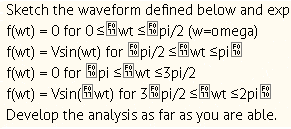Yes I will in the evening please dont remove it..

gneill
Mentor
gneill
Mentor
You have a piecewise-continuous function that's defined in several parts. Can you make a sketch of one full period (0 to ##2 \pi## radians). And maybe clarify what those garbled characters are in the function definition. Are they important?

gneill
Mentor
Okay, a couple of things before tackling the Fourier series. The first is a minor nit: In your function definition you have used ≤ for both of the segment ends where they transition from one segment to the other. This means that at the points where function changes from one segment to another the function would have two values (one from each segment) so it really wouldn't be a true function.

The second is that your sketch of the function doesn't look right. For example, the function should be zero until ωt is 90° (the first segment of the function definition) then it transitions to Vsin(ωt). Well, ωt is 90° at that instant, and Vsin(90°) = V, since sin(90°) = 1. In other words, your sketch should show a sharp jump from 0 to V at ωt = 90°. Similarly at 270° there should be another sharp transition but this time from 0 to -V, since sin(270°) = -1.

After you've sorted out your sketch, see if you can spot the overall function's period and then set up the integrals for the Fourier coefficients.

gneill
Mentor
Closer. Note that the transitions from horizontal section to sine curve section should be vertical!

Closer. Note that the transitions from horizontal section to sine curve section should be vertical!

I do not want to make myself look silly but please explain me is this first part shall I integrate over a period and achieve 1/pi x pi/2 and that gives me 1/2 at the begin of the function?

gneill
Mentor
I do not want to make myself look silly but please explain me is this first part shall I integrate over a period and achieve 1/pi x pi/2 and that gives me 1/2 at the begin of the function?
I'm not sure what you're asking here. My previous comment was to point out that the function makes a sudden transition from 0 to 1 at ωt = 90°, and from 0 to -1 at ωt = 270°. After the transitions the curve follows sin(ωt) until the next zero, where it goes back to a horizontal (zero) value for the next segment.

Perhaps you should plot a few points around the segment transitions to see.

## Homework Statement

Sketch the waveform defined below and explain how you would obtain its Fourier series:
f(wt) = 0 for 0 ≤wt ≤pi/2 (w=omega)
f(wt) = Vsin(wt) for pi/2 ≤wt ≤pi
f(wt) = 0 for pi ≤wt ≤3pi/2
f(wt) = Vsin(wt) for 3pi/2 ≤wt ≤2pi
Develop the analysis as far as you are able.

## The Attempt at a Solution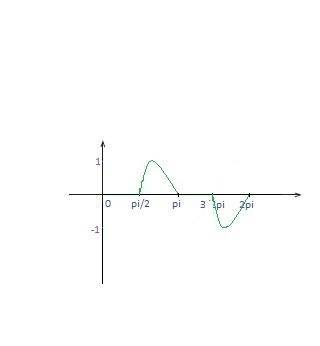a sudden transition from 0 to 1 at pi/2
same for 270 with minus 1

gneill
Mentor
f(wt) = 0 for 0 ≤ wt ≤ pi/2 (w=omega) ← at wt = ##\pi/2## f(wt) = 0
f(wt) = Vsin(wt) for pi/2 ≤ wt ≤pi ← at wt = ##\pi/2## f(wt) = sin(wt) = 1
f(wt) = 0 for pi ≤ wt ≤3pi/2 ← at wt = ##3 \pi/2## f(wt) = 0
f(wt) = Vsin(wt) for 3pi/2 ≤ wt ≤2pi ← at wt = ##3 \pi/2## f(wt) = sin(wt) = -1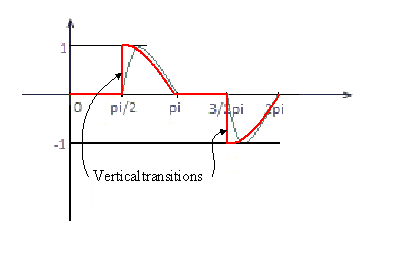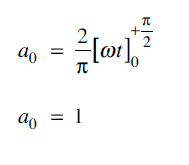Sir I have a question related to the picture above and also connected with thread how a0 is obtained in this particular example? is it something to do with cosine? I am not sure how a0 = 1 is calculated? can I ask for some hint in here?

gneill
Mentor
View attachment 78040
Sir I have a question related to the picture above and also connected with thread how a0 is obtained in this particular example? is it something to do with cosine? I am not sure how a0 = 1 is calculated? can I ask for some hint in here?
I don't see where that expression comes from. How did wt "escape" from its trig function? Can you show more of your work?

I don't see where that expression comes from. How did wt "escape" from its trig function? Can you show more of your work?
this what I am asking I was studying example to try to form some integrals and I did not understand how in this particular example a0 = 1 what is the process..?

gneill
Mentor
this what I am asking I was studying example to try to form some integrals and I did not understand how in this particular example a0 = 1 what is the process..?
I don't know how they got there since I can't see the full development.

When I worked through the problem as you've presented it I found an expression for an that yielded a0 = 0. Perhaps they framed the problem differently and thus arrived at a different result.

Can you please clarify something for me 1/∏ ∫ sin (wt) dwt can I "escape" to 1/pi [-cos wdt] and then "escape" to -eg 1/pi[ cos pi - cos pi/2] ??

I don't know how they got there since I can't see the full development.

When I worked through the problem as you've presented it I found an expression for an that yielded a0 = 0. Perhaps they framed the problem differently and thus arrived at a different result.
oh ok forget the last pic then that is just me trying to undersand whole concept. I started of calculating a0 and my final line is = 1/pi- 1/pi =0 so now next steps is an and bn ?

gneill
Mentor
oh ok forget the last pic then that is just me trying to undersand whole concept. I started of calculating a0 and my final line is = 1/pi- 1/pi =0 so now next steps is an and bn ?
Sure.

Sure.
Gneill I need some of your expertise on an ..
I was trying to figure it out =2/npi [sin suqare (npi)- sin square pi/2] + 2/npi [sin square (n2pi) - sin 3/2pi] anywhere near or shall I remove square ??gneill
Mentor
Gneill I need some of your expertise on an ..
I was trying to figure it out =2/npi [sin suqare (npi)- sin square pi/2] + 2/npi [sin square (n2pi) - sin 3/2pi] anywhere near or shall I remove square ??What do you mean by "figure it out"? Are you trying to simplify it some way?

Can I ask, for this question is a full Fourier series expected to be given? As the question says explain how u would obtain the F.S I expected just to explain what tests I would do on the waveform to check for coefficients and odd or even harmonic content etc.
Thanks

gneill
Mentor
Can I ask, for this question is a full Fourier series expected to be given? As the question says explain how [yo]u would obtain the F.S I expected just to explain what tests I would do on the waveform to check for coefficients and odd or even harmonic content etc.
Thanks
I would sketch the waveform, determine that it can be interpreted as a portion of a periodic function, indicate the appropriate period on the sketch, state whether that would be an even or odd function and any implications that might have on Fourier terms, and set up the integrals for the Fourier Transform but not necessarily solve them.

Does that help?

I would sketch the waveform, determine that it can be interpreted as a portion of a periodic function, indicate the appropriate period on the sketch, state whether that would be an even or odd function and any implications that might have on Fourier terms, and set up the integrals for the Fourier Transform but not necessarily solve them.

Does that help?
yes thank you! I think the function is neither odd nor even, applying f(-x) = f(x) and f(-x) = -f(x) In which both cases are untrue, I used 45 degrees as the value of x. Is this correct? I originally used 90 degrees as the X value in which I think wasn't a great value to use as the rule for an odd function was true. Thanks

gneill
Mentor
With a suitable coordinate shift you might find some symmetry to exploit...

Edit: Never mind. I misrecalled what the function sketch looked like. I went back and checked (post #15) and realized that the symmetry I was alluding to isn't actually there!I shouldn't rely so much on my memory for these important details...

BvU
Homework Helper
Hello all,

From the first clear picture of the function in post #15: This looks an awful lot like the product of two functions that are a little easier to transform: One is ##\sin \omega t##, the other a square wave.

This exercise is an opportunity to learn about the convolution theorem.
And consult some examples of Fourier transforms and - relationships

From the difficulties the OP has in getting an idea of the function I gather some more help on the way to the answer will be needed ...

With a suitable coordinate shift you might find some symmetry to exploit...

Edit: Never mind. I misrecalled what the function sketch looked like. I went back and checked (post #15) and realized that the symmetry I was alluding to isn't actually there!I shouldn't rely so much on my memory for these important details...
Am I right in saying then gneill that there is no symmetry and that applying the rules for odd and even functions this proves that?

As I mentioned I think what threw me is at + and - 90 degrees the rule for an odd function could be true but applying this at 45 degrees neither odd or even rule is true.

BvU
Homework Helper
Am I right in saying then gneill that there is no symmetry and that applying the rules for odd and even functions this proves that?
There is plenty of symmetry, just not the very simplest symmetry ##f(-x) = \pm f(x)##

gneill
Mentor
Am I right in saying then gneill that there is no symmetry and that applying the rules for odd and even functions this proves that?
I would agree that the test shows that the function is neither odd nor even.

I would agree that the test shows that the function is neither odd nor even.
Thanks both gneil and BvU :-)

Lordflasheart
Hi this is my first post please be gentle, I am on a track to answers to this question however my coefficient values seem different to this post and https://www.physicsforums.com/threads/fourier-series-of-a-waveform.766224/page-2#post-5143480.

Am I way off track with my understanding which I have attached for your attention.

Regards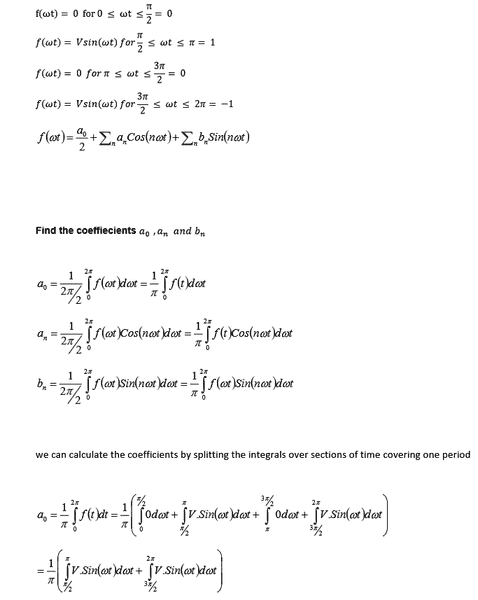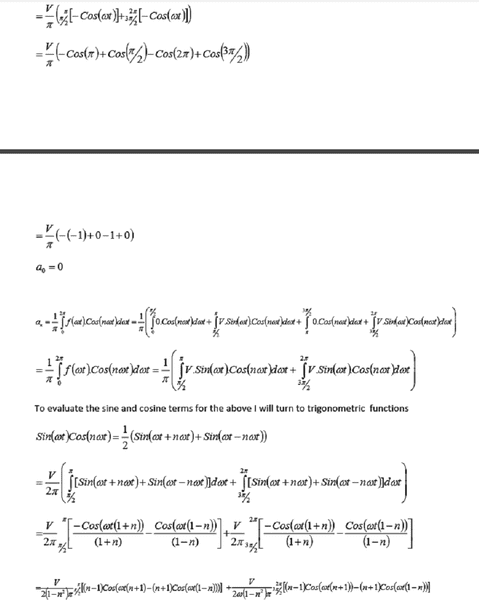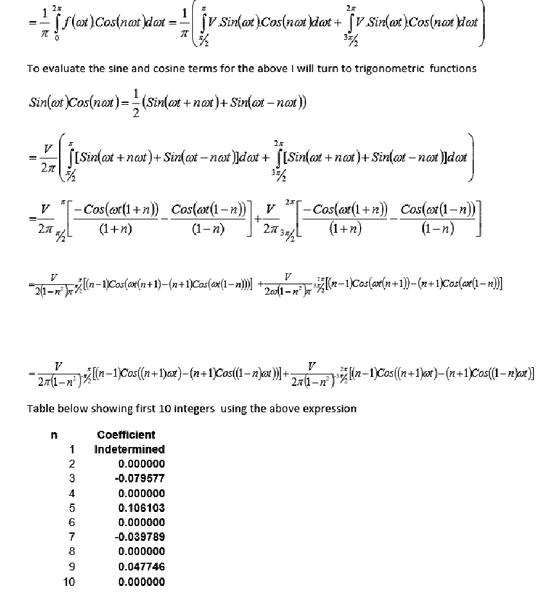#### Attachments

Last edited by a moderator: